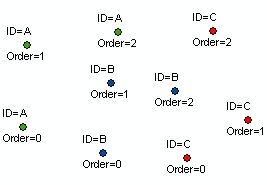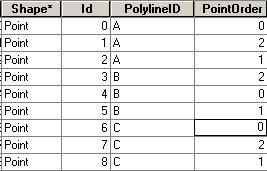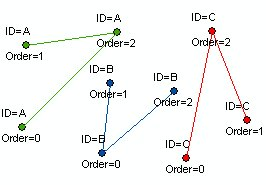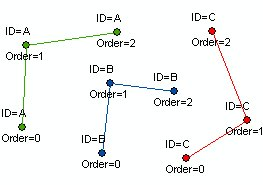Points To Polylines

Running programmatically

Converts a point data set to a polyline layer. Attaches to the polyline attribute table the values of the attributes for the first and last point that form a single polyline. If your point data does not have Polyline ID and Order attributes, you can try the Connect Unstructured Points function.

Inputs:

• A point feature layer
• REQUIRED: an ID field which value defines the points to be used for creation of each polyline
• OPTIONAL: an Order field that defines in what sequence the points describe the polyline. If no Order field is used the order is defined by the record number of the points
• OPTIONAL: a Link field. The values for the first and last point that will form a single polyline will be added to the polyline attribute table.
• OPTIONAL: Z Value field. If specified, a PolylineZ feature class will be created. The values in this field will be set as Z values for the vertices. If the input points have Z values, the user can specify the Z values of the input points to ve used by selecting "Features" for Z Value field.
• OPTIONAL: M Value field. If specified, a PolylineM feature class will be created. The values in this field will be set as M values for the vertices. If the input points have M values, the user can specify the M values of the input points to ve used by selecting "Features" for M Value field.

Outputs:

• New polyline layer
• Fields to be added to the polyline attribute table
• [ET_ID] - the field used as Polyline ID
• [ET_FromAtt] - the values of the start point of the polyline in the Link field (if link field is used)
• [ET_ToAtt] - the values of the end point of the polyline in the Link field (if link field is used)

Notes:

• There should be at least two points with the same value in the ID field in order polylines to be created.

Example:

Source pointsSource points attribute tableResulting polylines: ID Field = [PolylineID], No Order UsedResulting polylines: ID Field = [PolylineID], Order field = [PointOrder]Running Programmatically

(Go to TOP)

Parameters

Expression Explanation
Function Name PointsToPolylines
<input dataset> A String representing the input layer. Must be of Point type.
<output dataset> A String - the full name of the output layer.
<ID Field> A String - the name of the field which values will indicate the points used to form a single Polyline feature.
{Order Field} A String - the name of a Numeric (integer or double) field which values will indicate the order in which the points describe the polylines.If no Order field is used the order is defined by the record number of the points.
{Z Field} A String - the name of the field which values will be used for Z values of the vertices. "Features" can be used if the input points have Z.
{M Field} A String - the name of the field which values will be used for M values of the vertices. "Features" can be used if the input points have M.
{Link Field} A String - the name of a field to be used as a link between the input points and the output. The values for the first and last point that will form a single polyline will be added to the polyline attribute table.

Running the function

ETGWPath used in the table below is the  full path to ETGWRun.exe (E.G. "C:\Program Files\ETSpatial Techniques\ETGeo Wizards\ETGWRun.exe")

Language Syntax
Python subprocess.call([ETGWPath, "PointsToPolylines", "input dataset", "output dataset", "ID Field", "Order Field", "Z Field", "M Field","Link Field"])
.NET using ETGWRun.exe StartInfo.FileName = ETGWPath
StartInfo.Arguments = "PointsToPolylines" "input dataset" "output dataset" "ID Field" "Order Field" "Z Field" "M Field" "Link Field"
.NET using ETGWOutX.dll PointsToPolylines(input dataset, output dataset, ID Field, Order Field, Z Field, M Field,Link Field)
ArcPy arcpy.PointsToPolylines(input dataset, output dataset, ID Field, "Order Field", "Z Field", M Field, Link Field)

Notes:

• <> - required parameter

• {} - optional parameter

• See examples for Python , .NET or ArcPy

• The argument separator for StartInfo.Arguments is space. If a string might contain a space, you need to double quote it.

(Go to TOP)